# xgxr Overview

## Overview

The xgxr package supports a structured approach to exploring PKPD data (outlined here). It also contains helper functions for enabling the modeler to follow best R practices (by appending the program name, figure name location, and draft status to each plot) and enabling the modeler to follow best graphical practices (by providing an xgx theme that reduces chart ink, and by providing time-scale, log-scale, and reverse-log-transform-scale functions for more readable axes).

## Required Packages

library(xgxr)
library(ggplot2)
library(dplyr)

## Traceability: annotating and saving plots and tables

### Saving figures

Our best practices require that we mark plots as “DRAFT” if not yet final, and also list the program that created the plot and the location where the plot is stored. This helps with the traceability of the work, by ensuring that the following information is available for every plot in a report: the R script used to create the figure, the location where the figure is stored, and the time and date when the figure was created. The key functions here are: * xgx_annotate_status allows for the addition of text (like the word draft) to the plots * xgx_annotate_filenames allows for printing the filenames as a caption for the plot. It requires an input list dirs with particular fields, as shown below.

The function xgx_save calls both of the above functions and it is illustrated below.

This function also requires the user to input a width and height for the graph. This is because often, the plots that are created have font that is so small that it’s impossible to read the x and y axes. We’ve found that the easiest way to set the font size is “indirectly” by specifying the height and width of the graph. Note that if you have a plot window open, you can get the height and width by typing dev.size()

dirs <- list(
parent_dir = tempdir(),
rscript_dir = tempdir(),
rscript_name = "example.R",
results_dir = tempdir(),
filename_prefix = "example_")
data <- data.frame(x = 1:1000, y = stats::rnorm(1000))
g <- xgx_plot(data = data, aes(x = x, y = y)) +
geom_point()
xgx_save(width = 4, height = 4, dirs = dirs, filename_main = "example_plot", status = "DRAFT")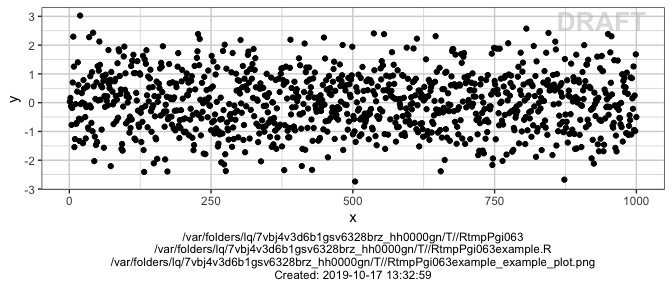The the function xgx_save works only with ggplot objects. If the figure that is created is not a ggplot object, it will not work. An alternative is to use xgx_annotate_status_png to add the status and filename to png files.

data <- data.frame(x = 1:1000, y = stats::rnorm(1000))
g <- xgx_plot(data = data, aes(x = x, y = y)) +
geom_point()
filename = file.path(tempdir(), "png_example.png")
ggsave(filename, plot = g, height = 4, width = 4, dpi = 75)
xgx_annotate_status_png(filename, "./ExampleScript.R")
#> Add footnote to /var/folders/lq/7vbj4v3d6b1gsv6328brz_hh0000gn/T//RtmpPgi063/png_example.png

### Saving tables

We also provide a function xgx_save_table for annotating the relevant information to csv files. The annotated table is shown below.

x <- data.frame(ID = c(1, 2), SEX = c("male", "female"))
data <- xgx_save_table(x, dirs = dirs, filename_main = "ExampleTable")
knitr::kable(data)
ID SEX
1 male
2 female

/var/folders/lq/7vbj4v3d6b1gsv6328brz_hh0000gn/T//RtmpPgi063
/var/folders/lq/7vbj4v3d6b1gsv6328brz_hh0000gn/T//RtmpPgi063/example.R
/var/folders/lq/7vbj4v3d6b1gsv6328brz_hh0000gn/T//RtmpPgi063/example_unnamed_table_.csv
Created: /2019-10-17 13:33:00

## Graphics helpers

### xgx theme

The xgx_theme() function includes the xGx recommended plot settings. It sets the background to white with light grey lines for the major and minor breaks. This minimizes chart ink as recommended by Edward Tufte. You can add xgx_theme() to an existing ggplot object, or you can call xgx_plot() in place of ggplot() for all of your plot initiations.

xgx_plot(mtcars, aes(x = cyl, y = mpg)) + geom_point()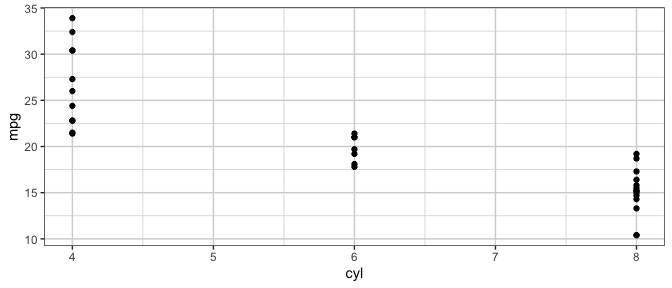You may wish to set the theme to xgx_theme for your R session, as we do below.

theme_set(xgx_theme())

## Alternative, equivalent function:
xgx_theme_set()
# time <- rep(seq(1,10),5)
# id <- sort(rep(seq(1,5), 10))
# conc <- exp(-time)*sort(rep(rlnorm(5),10))
#
# data <- data.frame(time = time, concentration  = conc, id = factor(id))
# xgx_plot() + xgx_geom_spaghetti(data = data, mapping = aes(x = time, y = concentration, group = id, color = id))
#
# xgx_spaghetti(data = data, mapping = aes(x = time, y = concentration, group = id, color = id))

### Confidence intervals

The code for confidence intervals is a bit complex and hard to remember. Rather than copy-pasting this code we provide the function xgx_stat_ci for calculating and plotting default confidence intervals. xgx_stat_ci allows the definition of multiple geom options in one function call, defined through a list. The default is geom = list("point","line","errorbar"). Additional ggplot options can be fed through the ggplot object call, or the xgx_stat_ci layer. (Note that xgx_stat_ci and xgx_geom_ci are equivalent)

data <- data.frame(x = rep(c(1, 2, 3), each = 20),
y = rep(c(1, 2, 3), each = 20) + stats::rnorm(60),
group = rep(1:3, 20))

xgx_plot(data,aes(x = x, y = y)) +
xgx_stat_ci(conf_level = .95)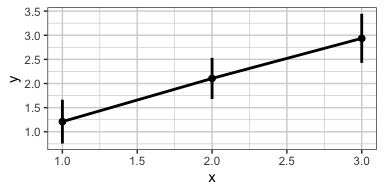xgx_plot(data,aes(x = x, y = y)) +
xgx_stat_ci(conf_level = .95, geom = list("pointrange","line"))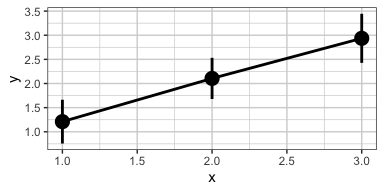xgx_plot(data,aes(x = x, y = y)) +
xgx_stat_ci(conf_level = .95, geom = list("ribbon","line"))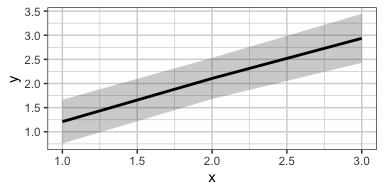xgx_plot(data,aes(x = x, y = y, group = group, color = factor(group))) +
xgx_stat_ci(conf_level = .95, alpha =  0.5,
position = position_dodge(width = 0.5))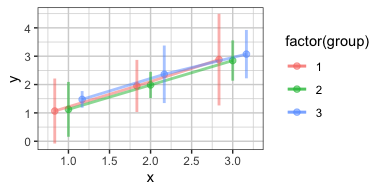The default settings calculate the confidence interval based on the Student t Distribution (assuming normally distributed data). You can also specify “lognormal”" or “binomial”" for the distribution. The former will perform the confidence interval operation on the log-scaled data, the latter uses the binomial exact confidence interval calculation from the binom package.

Note: you DO NOT need to use both distribution = "lognormal" and scale_y_log10(), choose only one of these.

# plotting lognormally distributed data
data <- data.frame(x = rep(c(1, 2, 3), each = 20),
y = 10^(rep(c(1, 2, 3), each = 20) + stats::rnorm(60)),
group = rep(1:3, 20))
xgx_plot(data, aes(x = x, y = y)) +
xgx_stat_ci(conf_level = 0.95, distribution = "lognormal")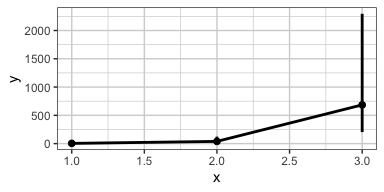# note: you DO NOT need to use both distribution = "lognormal" and scale_y_log10()
xgx_plot(data,aes(x = x, y = y)) +
xgx_stat_ci(conf_level = 0.95) + xgx_scale_y_log10()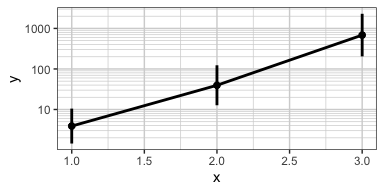# plotting binomial data
data <- data.frame(x = rep(c(1, 2, 3), each = 20),
y = rbinom(60, 1, rep(c(0.2, 0.6, 0.8), each = 20)),
group = rep(1:3, 20))
xgx_plot(data, aes(x = x, y = y)) +
xgx_stat_ci(conf_level = 0.95, distribution = "binomial")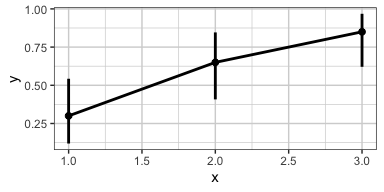### Nice log scale

This version of the log scale function shows the tick marks between the major breaks (i.e. at 1, 2, 3, … 10, instead of just 1 and 10). It also uses $10^x$ notation when the labels are base 10 and are very small or very large (<.001 or >9999)

df <- data.frame(x = c(0, stats::rlnorm(1000, 0, 1)),
y = c(0, stats::rlnorm(1000, 0, 3)))
xgx_plot(data = df, aes(x = x, y = y)) +
geom_point() +
xgx_scale_x_log10() +
xgx_scale_y_log10()
#> Warning: Transformation introduced infinite values in continuous x-axis
#> Warning: Transformation introduced infinite values in continuous y-axis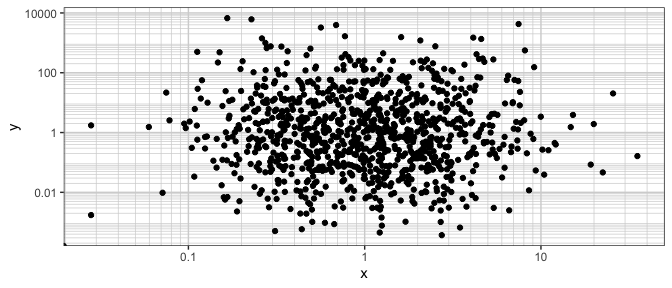### Reverse log transform

This transform is useful for plotting data on a percentage scale that can approach 100% (such as receptor occupancy data).

conc <- 10^(seq(-3, 3, by = 0.1))
ec50 <- 1
data <- data.frame(concentration = conc,
bound_receptor = 1 * conc / (conc + ec50))
gy <- xgx_plot(data, aes(x = concentration, y = bound_receptor)) +
geom_point() +
geom_line() +
xgx_scale_x_log10() +
xgx_scale_y_reverselog10()

gx <- xgx_plot(data, aes(x = bound_receptor, y = concentration)) +
geom_point() +
geom_line() +
xgx_scale_y_log10() +
xgx_scale_x_reverselog10()

gridExtra::grid.arrange(gy, gx, nrow = 1)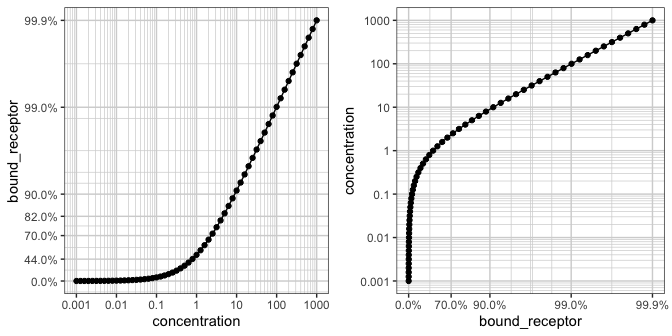### Scaling x-axis as a time scale

For time, it’s often good for the x ticks to be spaced in a particular way. For instance, for hours, subdividing in increments by 24, 12, 6, and 3 hours can make more sense than by 10 or 100. Similarly for days, increments of 7 or 28 days are preferred over 5 or 10 days. xgx_scale_x_time_units allows for this, where it is the input and output units.

data <- data.frame(x = 1:1000, y = stats::rnorm(1000))
g <- xgx_plot(data = data, aes(x = x, y = y)) +
geom_point()
g1 <- g + xgx_scale_x_time_units(units_dataset = "hours", units_plot = "hours")
g2 <- g + xgx_scale_x_time_units(units_dataset = "hours", units_plot = "days")
g3 <- g + xgx_scale_x_time_units(units_dataset = "hours", units_plot = "weeks")
g4 <- g + xgx_scale_x_time_units(units_dataset = "hours", units_plot = "months")

gridExtra::grid.arrange(g1, g2, g3, g4, nrow = 2)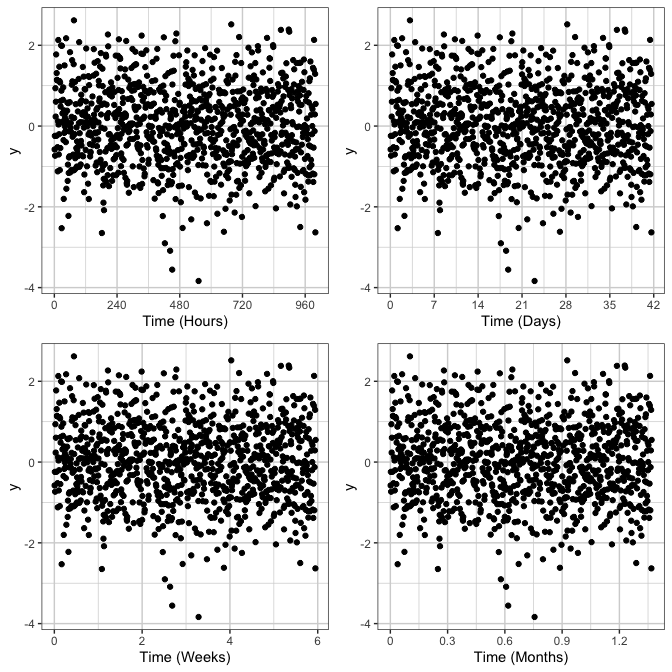## Data checking

### Numerical check

We’ve found that during exploration, it can be extremely important to check the dataset for issues. This can be done using the xgx_check_data or xgx_summarize_data function (the two functions are identical).

data <- mad_missing_duplicates %>%
filter(CMT %in% c(1, 2, 3)) %>%
rename(DV      = LIDV,
YTYPE   = CMT,
USUBJID = ID)
covariates <- c("WEIGHTB", "SEX")
check <- xgx_check_data(data, covariates)
#> Warning in xgx_check_data(data, covariates): Setting ID column equal to USUBJID
#>
#> DATA SUMMARY
#> CONTINUOUS COVARIATES
#> CATEGORICAL COVARIATES
#> POSSIBLE DATA ISSUES - FIRST 6 RECORDS
#> The following columns contained missing values
#> DV:368

knitr::kable(check$summary) Category Description YTYPE Statistic Value Patients Number of Patients - 60 60 MDV Number of patients with zero observations all 0 0 MDV Number of Missing Data Points (MDV==1 and EVID==0) 1 0 0 MDV Number of Missing Data Points (MDV==1 and EVID==0) 2 99 99 MDV Number of Missing Data Points (MDV==1 and EVID==0) 3 0 0 Dose Number of non-zero doses - 300 300 Dose Number of zero doses (AMT==0) - 60 60 Dose Number of patients that never received drug - 10 10 DV Number of Data Points 1 360 360 DV Number of Data Points 2 1309 1309 DV Number of Data Points 3 600 600 DV Number of Data Points per Individual 1 min = 6, median = 6, max = 6 6 DV Number of Data Points per Individual 2 min = 26, median = 26, max = 27 26 DV Number of Data Points per Individual 3 min = 10, median = 10, max = 10 10 DV Number of Data Points with zero value (DV==0) 1 0 0 DV Number of Data Points with zero value (DV==0) 2 0 0 DV Number of Data Points with zero value (DV==0) 3 0 0 DV Number of Data Points with NA (is.na(DV)) 1 0 0 DV Number of Data Points with NA (is.na(DV)) 2 8 8 DV Number of Data Points with NA (is.na(DV)) 3 0 0 DV+TIME Multiple measurements at same time 1 0 0 DV+TIME Multiple measurements at same time 2 32 32 DV+TIME Multiple measurements at same time 3 0 0 CENS Number of Censored Data Points 1 0 (0%) 0 CENS Number of Censored Data Points 2 9 (1%) 9 CENS Number of Censored Data Points 3 4 (1%) 4 All Columns Negative Values (number) - DV:12 12 All Columns Missing Values (number) - DV:368 368 knitr::kable(head(check$data_subset))
Data_Check_Issue ID TIME DV CENS YTYPE
is.na(DV) 15 0.000 NA 0 2
is.na(DV) 21 127.858 NA 0 2
is.na(DV) 22 216.537 NA 0 2
is.na(DV) 40 156.563 NA 0 2
is.na(DV) 47 120.300 NA 0 2
is.na(DV) 50 71.814 NA 0 2

You can also get an overview of the covariates in the dataset with xgx_summarize_covariates. The covariate summaries are also provided in the xgx_check_data and xgx_summarize_data functions.

covar <- xgx_summarize_covariates(data,covariates)
knitr::kable(covar$cts_covariates) Covariate Nmissing min 25th median 75th max WEIGHTB 0 52.8 69.2 78.9 89.85 109 knitr::kable(covar$cat_covariates)
Covariate Nmissing Ndistinct Value (Count)
SEX 0 2 Female (30), Male (30)